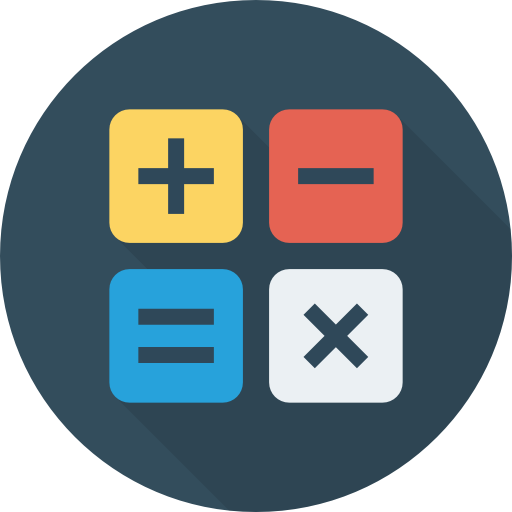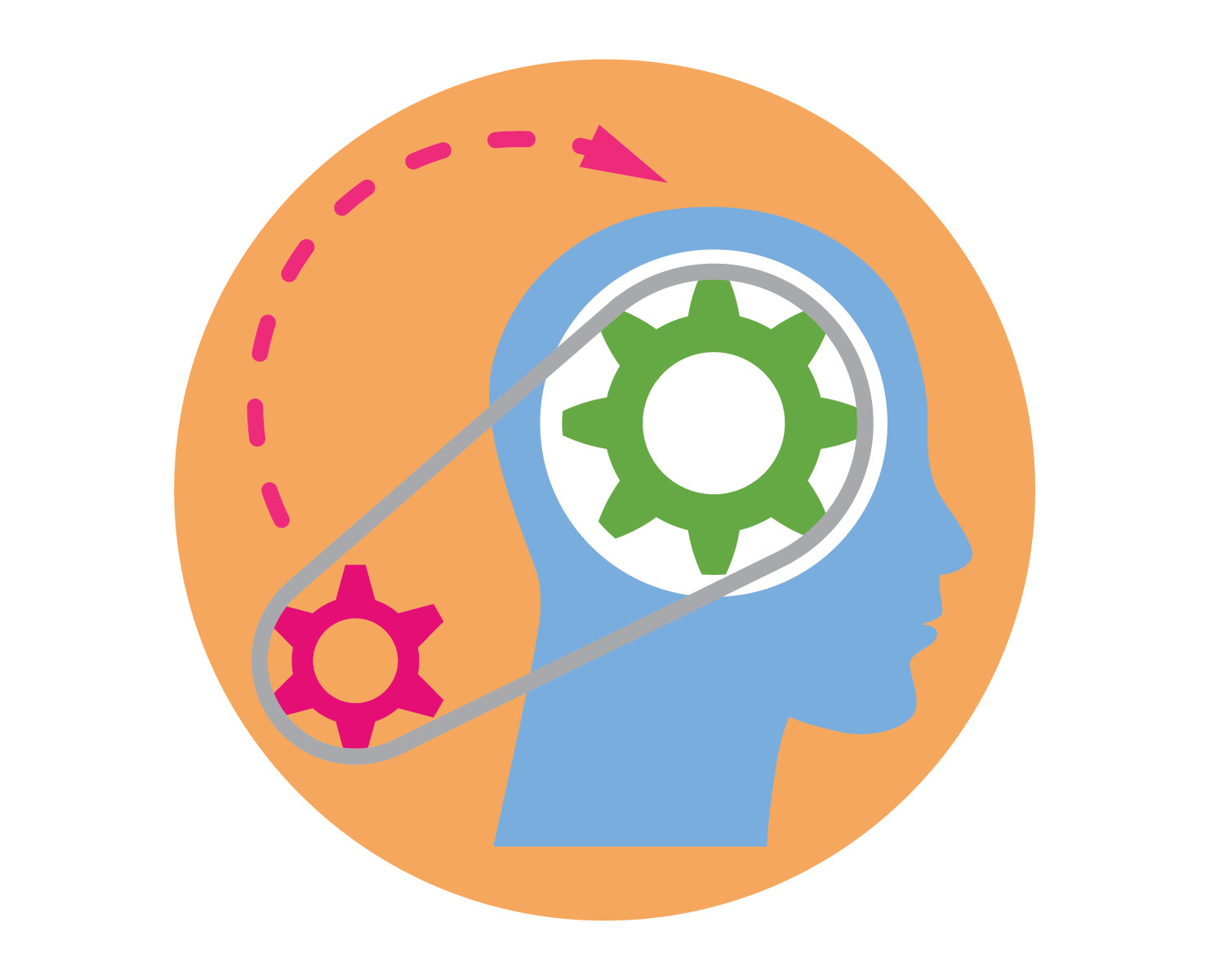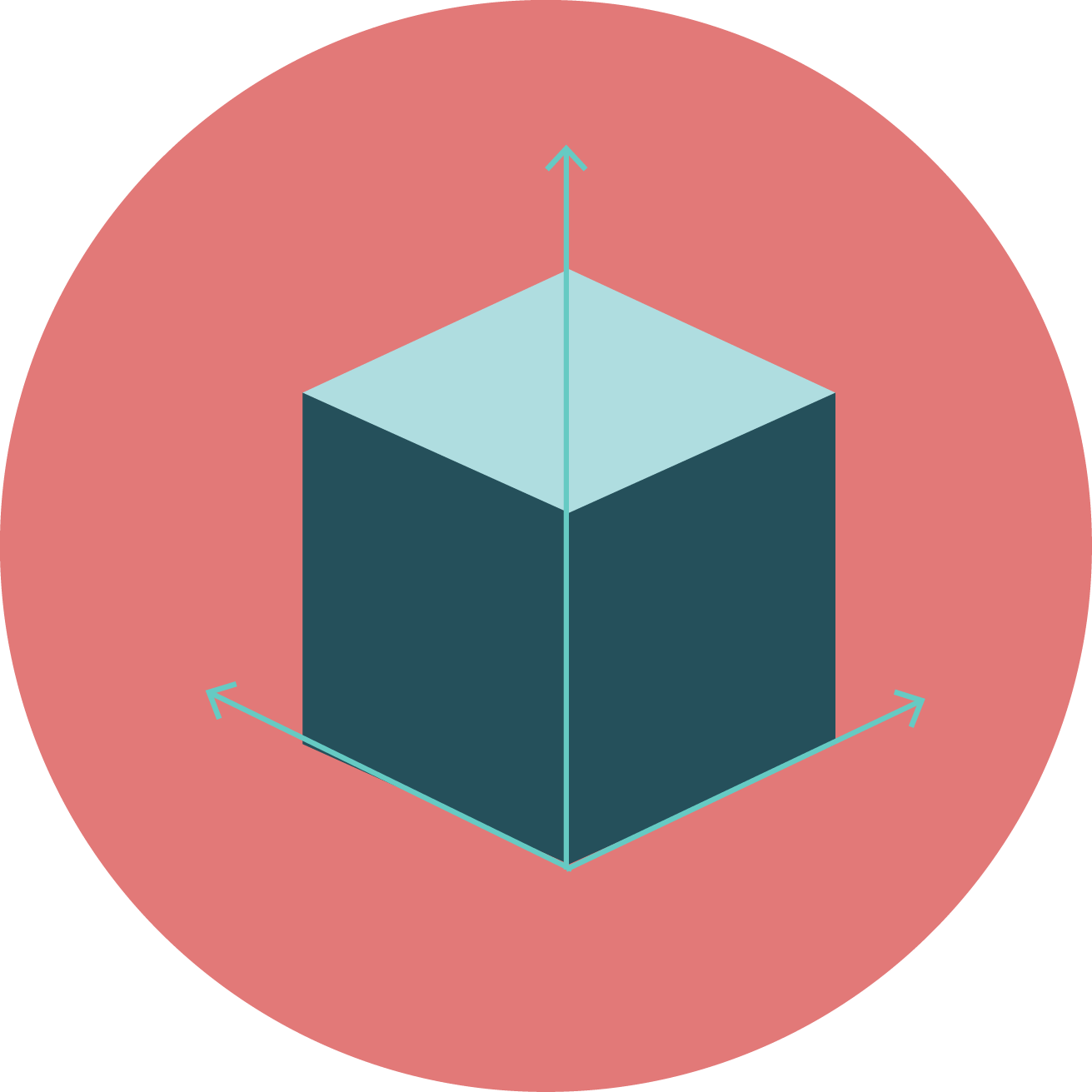## OGO EXAMINATION (SLE)

### MATHEMATICS

#### Skills Assessed

##### Arithmetical##### ProblemSolving##### Logical Reasoning##### Spatial Understanding##### DataInterpretation#### Exam Pattern

• Total 30 Questions
• 5 questions based on Logical Reasoning
• 20 questions based on Subject Knowledge
• 5 questions based on competency
• Total 40 questions
• 8 questions based on Logical Reasoning
• 20 questions based on Subject Knowledge
• Out of these 40 questions, 5 questions will have 2-key answers
• 7 questions bases on competency
• Total 50 questions
• 10 questions based on Logical Reasoning
• 25 questions based on Subject Knowledge
• Out of these 50 questions, 5 questions will have 2-key answers
• 10 questions based on competency

#### Curriculum

Chapters

Numbers; Addition; Subtraction; Comparison; Measurement; Time and Calendar; Money; Shapes; Patterns; Data Handling

Competency Enhancement

Same as chapters

Logical Reasoning

Critical Thinking, Analysis, Shapes Patterns, Puzzle, Decoding, Pictorial

Chapters

Numbers; Addition; Subtraction; Multiplication; Division; Measurement; Time and Calendar; Money; Shapes; Patterns; Data Handling

Competency Enhancement

Same as chapters

Logical Reasoning

Critical Thinking, Analysis, Shapes and Patterns, Puzzle, Decoding, Pictorial

Chapters

Numbers; Addition; Subtraction; Multiplication; Division; Fractions; Measurement; Time and Calendar; Money; Shapes; Patterns; Data Handling

Competency Enhancement

Same as chapters

Logical Reasoning

Critical Thinking, Analysis, Shapes and Patterns, Puzzle, Decoding, Pictorial

Chapters

Numbers; Addition and Subtraction; Multiplication and Division; Factors and Multiples; Fractions; Decimals; Measurement and Money; Time and Calendar; Geometrical Figures; Perimeters and Areas; Patterns and Symmetry; Data Handling

Competency Enhancement

Same as chapters

Logical Reasoning

Critical Thinking, Analysis, Shapes and Patterns, Puzzle, Decoding, Pictorial

Chapters

Large Numbers; Four Operations; LCM and HCF; Fractions; Decimals; Measurement and Money; Time and Calendar; Basic Geometry; Perimeters and Areas; Solid Figures and Volumes; Patterns and Symmetry; Data Handling

Competency Enhancement

Same as chapters

Logical Reasoning

Critical Thinking, Analysis, Shapes and Patterns, Puzzle, Decoding, Pictorial

Chapters

Number System; Whole Numbers; Playing with Numbers; Geometry; Integers; Fractions; Decimals;

Mensuration; Algebra; Ratio and Proportion; Data Handling; Symmetry and Practical Geometry

Competency Enhancement

Same as chapters

Logical Reasoning

Critical Thinking, Analysis, Shapes and Patterns, Puzzle, Decoding, Pictorial

Chapters

Integers; Fractions and Decimals; Data Handling; Simple Equations; Lines and Angles; The Triangle and its Properties; Congruence of Triangles; Comparing Quantities; Rational Numbers; Practical Geometry; Perimeter and Area; Algebraic Expressions; Exponents and Powers; Symmetry and Visualising Solid Shapes

Competency Enhancement

Same as chapters

Logical Reasoning

Critical Thinking, Analysis, Shapes and Patterns, Puzzle, Decoding, Pictorial

Chapters

Rational Numbers; Linear Equations in One Variable; Understanding Quadrilaterals; Practical Geometry; Data Handling; Squares and Square Roots; Cubes and Cube Roots; Comparing Quantities; Algebraic Expressions and Identities; Visualising Solid Shapes; Mensuration; Exponents and Powers; Direct and Inverse Proportions; Factorisation; Introduction to Graphs; Playing with Numbers

Competency Enhancement

Same as chapters

Logical Reasoning

Critical Thinking, Analysis, Shapes and Patterns, Puzzle, Decoding, Pictorial

Chapters

Number System; Polynomials; Coordinate Geometry; Linear Equations in Two Variables; Introduction to Euclid’s Geometry; Lines and Angles; Triangles; Quadrilaterals; Areas of Parallelograms and Triangles; Circles; Constructions; Heron’s Formula; Areas and Volumes; Statistics and Probability

Competency Enhancement

Same as chapters

Logical Reasoning

Critical Thinking, Analysis, Shapes and Patterns, Puzzle, Decoding, Pictorial

Chapters

Real Numbers; Polynomials; Pair of Linear Equations in Two Variables; Quadratic Equations; Arithmetic Progressions; Triangles; Coordinate Geometry; Introduction to Trigonometry; Some Applications of Trigonometry; Circles; Constructions; Areas Related to Circles; Surface Areas and Volumes; Statistics; Probability

Competency Enhancement

Same as chapters

Logical Reasoning

Critical Thinking, Analysis, Shapes and Patterns, Puzzle, Decoding, Pictorial